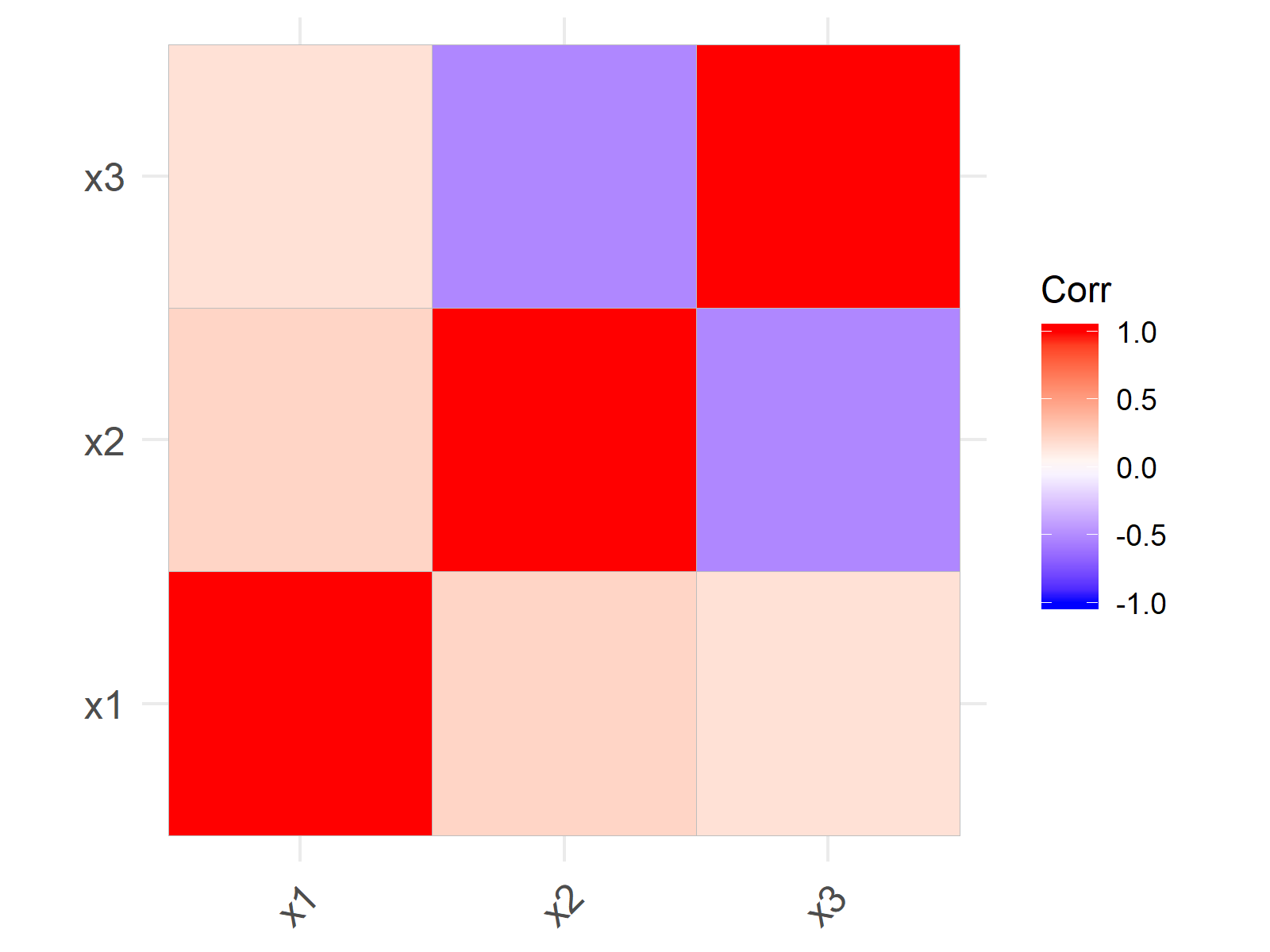# Correlation Matrix in R (3 Examples)

In this tutorial you’ll learn how to compute and plot a correlation matrix in the R programming language.

The article consists of three examples for the creation of correlation matrices. More precisely, the article looks as follows:

So let’s dive right into the programming part.

## Example Data

I’ll use the data below as basement for this R tutorial:

```set.seed(28762)                           # Create example data
x1 <- rnorm(1000)
x2 <- rnorm(1000) + 0.2 * x1
x3 <- runif(1000) + 0.1 * x1 - 0.2 * x2
data <- data.frame(x1, x2, x3)
#            x1         x2        x3
# 1 -0.18569232 -0.9497532 1.0033275
# 2  0.28981164 -0.9131415 0.7393190
# 3 -1.76015009 -2.1335438 1.1012058
# 4  0.01030804 -0.4538802 0.3128903
# 5  0.43926986 -0.2940416 0.1996600
# 6 -2.25920975 -0.4394634 0.1017577```

As you can see based on the previous output of the RStudio console, our example data contains three numeric variables.

## Example 1: Compute Correlations Between Variables

Example 1 explains how to calculate the correlation values between each pair of columns of a data set.

```cor(data)                                 # Correlation matrix of example data
#           x1         x2         x3
# x1 1.0000000  0.2225584  0.1625305
# x2 0.2225584  1.0000000 -0.5150919
# x3 0.1625305 -0.5150919  1.0000000```

As you can see based on the previous output of the RStudio console, we created a matrix consisting of the correlations of each pair of variables. For instance, the correlation between x1 and x2 is 0.2225584.

## Example 2: Plot Correlation Matrix with corrplot Package

The R syntax below explains how to draw a correlation table in a plot with the corrplot package.

First, we need to install and load the corrplot package, if we want to use the corresponding functions:

```install.packages("corrplot")                      # Install corrplot package

Now, we can use the corrplot function as shown below:

`corrplot(cor(data), method = "circle")    # Apply corrplot function`As visualized in Figure 1, the previous R programming syntax created a correlation matrix graphic indicating the size of the correlation with colored circles.

## Example 3: Plot Correlation Matrix with ggcorrplot Package

This Example explains how to plot a correlation matrix with the ggcorrplot package. The ggcorrplot package is part of the ggplot2 family.

```install.packages("ggcorrplot")                      # Install ggcorrplot package

Now, we can use the ggcorrplot to create a correlation graph in the style of the ggplot2 package.

`ggcorrplot(cor(data))                     # Apply ggcorrplot function`As revealed in Figure 2, we created a correlation matrix plot with the previous R programming syntax.

## Video & Further Resources

Do you want to learn more about the computation and plotting of correlations? Then you may want to have a look at the following video of my YouTube channel. In the video, I illustrate the R codes of the present article:

Please accept YouTube cookies to play this video. By accepting you will be accessing content from YouTube, a service provided by an external third party.If you accept this notice, your choice will be saved and the page will refresh.

Furthermore, you may have a look at the other posts of my website. A selection of other articles is shown here.

This tutorial explained how to get a matrix table containing Pearson correlation coefficients in the R programming language. Please let me know in the comments section, in case you have additional questions. In addition, please subscribe to my email newsletter to get updates on the newest tutorials.

Subscribe to the Statistics Globe Newsletter

•Pharaoh Fellow Mwale
August 20, 2022 9:18 am

This tutorial helped me a lot to understand the correlation plot in R. Thank you very much for the tutorial. However, I want to know how to program with R to draw a RRHo plot. I would like to determine some genes that are expressed in the brain and how they are correlated when treated in terms of fold change and in terms of log scale. Would you help me, thank you in advance.

•August 22, 2022 8:58 am